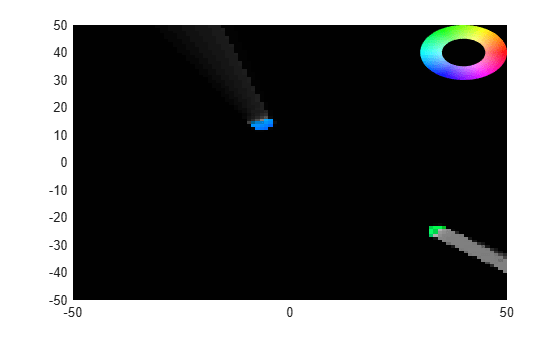# dynamicEvidentialGridMap

Dynamic grid map output from `trackerGridRFS`

Since R2021a

## Description

The `dynamicEvidentialGridMap` object represents the dynamic map estimate obtained from the grid-based tracker, `trackerGridRFS`. You can visualize the dynamic map and obtain the estimated values by using the object functions of `dynamicEvidentialGridMap`. The `dynamicEvidentialGridMap` object is a handle object.

## Creation

You can generate a `dynamicEvidentialGridMap` object using a `trackerGridRFS` object. See the Usage syntax of `trackerGridRFS` and the Obtain Estimated Values at Grid Level using `dynamicEvidentialGridMap` example for details.

## Properties

expand all

Motion model for tracking, specified as `'constant-velocity'`, `'constant-acceleration'`, or `'constant-turn-rate'`. The particle state and object state for each motion model are:

`MotionModel`Particle StateObject State
`'constant-velocity'``[x; vx; y; vy] ``[x; vx; y; vy; yaw; L; W]`
`'constant-acceleration'``[x; vx; ax; y; vy; ay]``[x; vx; ax; y; vy; ay; yaw; L; W]`
`'constant-turn-rate'``[x; vx; y; vy; w]``[x; vx; y; vy; w; yaw; L; W]`

where:

• `x` — Position of the object in the x direction of the tracking frame (m)

• `y` — Position of the object in the y direction of the tracking frame (m)

• `vx` — Velocity of the object in the x direction of the tracking frame (m/s)

• `vy` — Velocity of the object in the y direction of the tracking frame (m/s)

• `ax` — Acceleration of the object in the x direction of the tracking frame (m/s2)

• `ay` — Acceleration of the object in the y direction of the tracking frame (m/s2)

• `w` — Yaw-rate of the object in the tracking frame (degree/s)

• `yaw` — Yaw angle of the object in the tracking frame (deg)

• `L` — Length of the object (m)

• `W` — Width of the object (m)

Number of state variables corresponding to the motion model specified in the `MotionModel` property, specified as a positive integer.

Example: `6`

x-direction dimension of the grid in the local coordinates, specified as a positive scalar in meters.

y-direction dimension of the grid in the local coordinates, specified as a positive scalar in meters.

Resolution of the grid, specified as a positive scalar. `GridResolution` represents the number of cells per meter of the grid for both the x- and y-direction of the grid.

Location of the grid origin in the local coordinate frame, specified as a two-element real-valued vector in meters. The grid origin represents the bottom-left corner of the grid map.

## Object Functions

 `getEvidences` Get estimated occupancy and free evidences `getOccupancy` Get estimated occupancy probabilities `getState` Get full estimated state and associated uncertainty `getVelocity` Get estimated velocity and associated uncertainty `show` Visualize dynamic evidential grid map

## Examples

collapse all

Create a tracking scenario.

```rng(2021);% For reproducible results scene = trackingScenario('UpdateRate',5,'StopTime',5);```

Add a platform. Mount a lidar sensor on the platform.

```plat = platform(scene); lidar = monostaticLidarSensor(1,'DetectionCoordinates','Body');```

Add two targets and define their position, velocity, orientation, dimension, and meshes.

```for i = 1:2 target = platform(scene); x = 50*(2*rand-1); y = 50*(2*rand-1); vx = 5*(2*rand-1); vy = 5*(2*rand-1); target.Trajectory.Position = [x y 0]; target.Trajectory.Velocity = [vx vy 0]; target.Trajectory.Orientation = quaternion([atan2d(vy,vx),0,0],'eulerd','ZYX','frame'); target.Mesh = extendedObjectMesh('sphere'); target.Dimensions = struct('Length',4, ... 'Width',4, ... 'Height',2, ... 'OriginOffset',[0 0 0]); end```

Define the configuration of the sensor.

```config = trackingSensorConfiguration(1, ... 'SensorLimits',[-180 180;0 100], ... 'SensorTransformParameters',struct, ... 'IsValidTime',true);```

Create a grid-based tracker.

```tracker = trackerGridRFS('SensorConfigurations',config, ... 'AssignmentThreshold',5, ... 'MinNumCellsPerCluster',4, ... 'ClusteringThreshold',3);```

Advance scenario and run the tracker based on the lidar data.

```while advance(scene) % Current time time = scene.SimulationTime; % Generate point cloud tgtMeshes = targetMeshes(plat); [ptCloud, config] = lidar(tgtMeshes, time); % Format the data for the tracker sensorData = struct('Time',time, ... 'SensorIndex',1, ... 'Measurement',ptCloud', ... 'MeasurementParameters',struct ... ); % Call tracker using sensorData to obtain the map in addition % to tracks [tracks, ~, ~, map] = tracker(sensorData,time); % Obtain the estimated occupancy probability of each cell P_occ = getOccupancy(map); % Obtain the estimated evidences for each cell [m_occ, m_free] = getEvidences(map); % Obtain the estimated velocity for each cell [v, Pv] = getVelocity(map); % Obtain the estimated state for each cell [x, P] = getState(map); % Show the map show(map,'InvertColors',true) end```## Version History

Introduced in R2021a